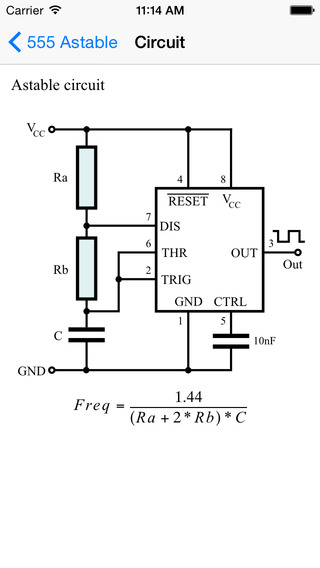# EE CalculatorThis app is a collection of basic electronic calculators. It is suitable for hobbyist, electronic engineers or professionals.Features• Calculate resistance of 2 resistors in series / parallel• To find out combinations of 2 resistors in series / in parallel making a desired resistance• Calculate ratio of voltage divider and find out combinations of resistor making a desired ratio• Calculate inverting amplifier gain and find out combinations of resistor making a desired ratio• Ohm's Law calculation• Star-delta conversion• RC charging circuit : Calculate time constant by R and C• RC Filter : Calculate cutoff frequency by resistor and capacitor value and find out combinations of resistance and capacitance making a desired cutoff frequency• RL Filter : Calculate cutoff frequency by resistor and inductor value and find out combinations of resistance and inductance making a desired cutoff frequency• LC Filter : Calculate resonant frequency by capacitor and inductor values and find out combinations of capacitance and inductance making a desired resonant frequency• Calculate monostable circuit• Calculate frequency of astable circuit and find out combinations of capacitor and resistor making a desired astable frequency • Save combinations in CSV file• Upload CSV file to Dropbox### 免費玩EE Calculator APP玩免費

#### 免費玩EE Calculator App

App Store
2.4.0
App下載

2014-03-062015-06-03